occasional meanderings in physics' brave new world

## Monday, June 30, 2008

### M Theory Lesson 203

As usual Carl has jumped ahead with a post on mixing matrices as magic squares. For reference, let us collect here some actual figures for the CKM matrix, given in this article from the Particle Data Group. Absolute value signs are omitted.

$M_{ud} = 0.97377 \pm 0.00027$
$M_{us} = 0.2257 \pm 0.0021$
$M_{ub} = 4.31 \pm 0.30 \times 10^{-3}$
$M_{cd} = 0.230 \pm 0.011$
$M_{cs} = 0.957 \pm 0.017 \pm 0.093$
$M_{cb} = 41.6 \pm 0.6 \times 10^{-3}$
$M_{td} = 7.4 \pm 0.8 \times 10^{-3}$
$M_{ts} = 40.6 \pm 2.7 \times 10^{-3}$
$M_{tb} > 0.78$

This is a little different to the values given in the wikipedia article. Standard Model analyses of these quantities can be quite complicated. Following the notation from before, in a very simple ideal double circulant the magic square property demands that $a + b = c + d$. For the CKM values (squared) we see that rows and columns do indeed sum to 1, and $c + d \simeq 1$ because $b$ is so small.

## Friday, June 27, 2008

### M Theory Lesson 202

So combining 1-circulants and 2-circulants is interesting in the context of mixing. Let us play with combinations of the Fourier operators for both cases. For example,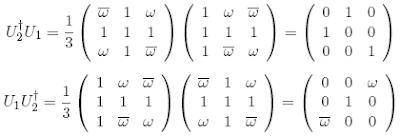Recall that these two matrices can be combined to describe an element of $B_{3}$ using a representation linked to the theory of the field with one element. We obtainwhich is a Bilson-Thompson type braid for particles. Since $U_{2}^{\dagger} U_{1} = U_{1}^{\dagger} U_{2}$, we can rewrite the quadruple product as $U_{1}^{\dagger} ( U_{2} U_{1} U_{2}^{\dagger} )$.

### Neutrinos Again VI

Let's get back to neutrino mixing. Today, Carl Brannen links to some slides by Smirnov on Universality versus Complementarity for quarks and leptons. Complementarity is the observation that for tribimaximal mixing one has the relations

$\theta_{12} + \theta_{12}^{qu} \simeq \frac{\pi}{4}$
$\theta_{23} + \theta_{23}^{qu} \simeq \frac{\pi}{4}$

(and $\theta_{13}$ small) despite the fact that the CKM matrix is very different to tribimaximal. Smirnov then discusses an implied $\nu_{\mu}$, $\nu_{\tau}$ permutation symmetry of the formBut observe that this matrix can also be expressed as the sum of two (Hermitian) $3 \times 3$ circulants, since 2-circulants describe the 2-cycles in the permutation group $S_{3}$.As a formal combination of elements of $S_{3}$ (or the braid group $B_{3}$ if appropriate phases are added) we can represent this matrix sum as an element of a diagram algebra on three strands.

### Phoenix

CV pointed out that the Phoenix Mars Lander has a Facebook account, so now I'm a friend of Phoenix! Today Phoenix told us that
the Martian soil is certainly friendly for life! Its pH is between 8 and 9 and quite salty!
I also became a fan of GLAST on Facebook.

## Thursday, June 26, 2008

### The Institute

Ben Webster asks us where and how we would build a new research institute. As previously mentioned, I would build a centre for pure Category Theory and its applications in everything from physics and computer science to neuroscience and linguistics. John Armstrong has already signed up for an NZ based institute. The first question is, urban or rural? Come on. This is the 21st century, so let's choose somewhere pleasant to live, where time off from the office can be spent on a variety of outdoor activities. Kaikoura is the place. Lonely Hapuku hutis only a few hours walk from potential institute sites in the lower Hapuku valley. There is access to a large area of scenic mountain hiking. One could take an easy walk up the Mt Fyfferoad in the morning and then enjoy whale watching in the afternoon.If tired of swimming with the seals, or skiing, or wine tasting, one could always turn to a geological tour. The so called Kaikoura orogeny, beginning about 25 million years ago, is the uplifting process that forms the Southern Alps. This institute would be cheap to build, since Kaikoura is sparsely populated. It is easily accessible by road and rail from Christchurch, three hours away.## Wednesday, June 25, 2008

### M Theory Lesson 201

It is confusing that we sometimes talk about 0, 1 and 2, and sometimes about 1, 2 and 3, when we really mean the same thing. But one denotes truth values by the former triple and elements of sets by the latter, even if the set contains the number 0. Of course it doesn't matter what one calls objects in a logos, so long as one is careful to explain what structure is being described. Let's stick with 1, 2 and 3 today, because this is conventional notation for the permutations on three objects, given as usual by the $3 \times 3$ circulant matrices with entries 0 and 1.

In logos theory multicategories are more important than ordinary categories, not least because operads are examples of multicategories. Consider the basic triangle category, with only three non-identity arrows. If the triangle is viewed as a multicocategory, what arrows can we draw with it? Any number of inputs is allowed, but for the category 3 repeats soon become inevitable. Heavy use of the identity arrows is made.Now consider a triangle with two way arrows between distinct objects. A pair of two way arrows can represent a 2-cycle permutation on two objects, denoted by the Pauli matrix $\sigma_{x}$. But then naive composition of arrows does not give the composition of 2-cycles in $S_{3}$. To obtain such a 3-cycle it is more natural to involve multiarrows! That is, let a trivalent vertex represent the 3-cycle, as we often do in M Theory.

## Saturday, June 21, 2008

### Foggy Friday

I live on a hill only about 250m above the low lying Canterbury plains, in fact on the other side of one of the low hills in the centre of this picture, on the outskirts of the city. But many winter mornings it is like living in the sky (although unfortunately it is often brown). Yesterday the fog sat even lower than in this (stolen) picture, and from home I could see across 100s of kilometres of cloud from a clear sunny day.## Friday, June 20, 2008

### M Theory Lesson 200

Now imagine that $\omega$ is an $N$th root of unity for some initially arbitrary $N$. Then our generators $\sigma_{1}$ and $\sigma_{2}$ obey ordinary matrix relations of the formand $\sigma_{1} \sigma_{2} \sigma_{1} = \sigma_{2} \sigma_{1} \sigma_{2}$ holds. We also have $(\sigma_{1} \sigma_{2} \sigma_{1})^{2} = \omega^{6} \cdot 1$, so if $\omega$ is a 6th root of unity the modular relation holds. One also has that $(\sigma_{1} \sigma_{2})^{3} = \omega^{6} \cdot 1$. This is the operator usually chosen to represent $ST$ in the modular group.

### M Theory Lesson 199

Let $\omega$ be the primitive cubed root of unity. Using the ordinary matrix product one finds that the prospective $B_3$ braid generatorsatisfies $\sigma_{2}^{2} = 1$, but as Lieven points out one can consider fancier matrix products, such asand it follows that instead $\sigma_{2}^{3}$ might be a permutation matrix. Anyhow, one easily verifies that the braid relation $\sigma_{1} \sigma_{2} \sigma_{1} = \sigma_{2} \sigma_{1} \sigma_{2}$ holds. Moreover, in this case of cubed roots of unity, using ordinary matrix product one gets the relation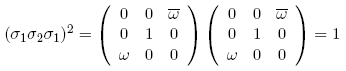which reduces the braid group to the modular group. Recall that this process views the group $B_{3}$ as the fundamental group of the complement of the trefoil knot in three dimensional space. Note that the generator $\sigma_{1}$ behaves similarly, reduced by the properties of $\omega$, but never quite to the identity. For powers of $\sigma_{1}$ we have the relations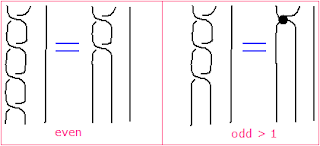where the big dot means the permutation operation, which has no knowledge of the crossing. What a nice way of looking at the modular group! Category theorists have a fancy way of thinking of semidirect products as a piece of two dimensional group structure, but these simple matrices are enough to see what is going on.

## Thursday, June 19, 2008

### Idempotent Nilpotent II

A nicer way to represent the neutrino tetrahedron group with $3 \times 3$ operators is to choose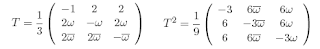since this also obeys $T^{3} = 1$ and $(TS)^{3} = 1$ but $T$ looks a lot more like the circulant $S$ than a diagonal operator. Moreover, the quantum Fourier diagonal still appears in the relation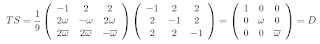from which it also follows that $T = SD$ and $T^{2} = S \overline{D} = S D^{2}$ where $\overline{D}$ is just the rotation in the opposite direction in the plane. Observe how the squaring of $T$ shifts the horizontal phase factors to vertical ones.

### Idempotent Nilpotent

Some circulants that pop up in M Theory have very nice properties. For example, consider the idempotent operator $\frac{1}{3} C$ inIf we were doing arithmetic modulo 3 this would look like the equationmaking the democratic matrix into a nilpotent operator. For a phase of $\delta = \pi$ the eigenvalues of $C$ are $(0, \sqrt{3}^{-1}, \sqrt{3}^{-1})$, which may be normalised to $(0,1,1)$. Note that, modulo 3, $C$ is the same as the modular operator $S$, which squares to unity and represents inversion in the unit circle. Modulo 2, the operator $C$ is the complement of the identity $S$.

### M Theory Lesson 198

Let's start with $(\mathbb{R}, \mathbb{C}, \mathbb{H})$ and the triple of Riemann surface moduli $(M(0,6), M(1,3), M(2,0))$, which have Euler characteristics $-6, - \frac{1}{6}, - \frac{1}{120}$ respectively. Observe that 120 is the number of elements in the icosahedral group, whereas 6 is the number of elements in $S_3$.

The triple of (orthogonal, unitary, symplectic) appeared in Mulase-Waldron T duality for partition functions over twisted graphs. Here, the unitary case is self dual, just like the Platonic tetrahedron. The real (orthogonal) case has half the number of matrix dimensions (punctures) as the quaternionic case, suggesting we associate the genus 1 moduli to $\mathbb{R}$ and the genus 0 moduli to $\mathbb{H}$. The dual graph to the cube is basically the 6 punctured sphere. This leaves the genus 2 moduli for the icosahedron and indeed the 120 in the Euler characteristic suggests a relation. Observe that without the octonions, one does not naturally encounter nonassociative structures in the triples, but such triples are also highly relevant to M Theory.

From a categorical perspective, one views these trinities as models of the category 3, the basic triangle, because they naturally form categories with only 3 objects and one natural map between any two objects. The collection of all such sets of three elements is the object 3 as an ordinal which counts cardinalities of sets, except that we have categorified the sets by making them categories! This is why it is not surprising to encounter grouplike cardinalities in the Euler characterstics of these models. (Actually, it is the orbifold structure of the moduli that gives them a groupoid character).

## Wednesday, June 18, 2008

### Lieven's Trinities

Lieven Le Bruyn has an absolutely wonderful post about Arnold's trinities. Examples include the Platonic groups, the exceptional triple $(E_6, E_7, E_8)$ and the fields $\mathbb{C}$, $\mathbb{H}$ and the octonions. Lieven asks, do you have other trinities you like to worship?

In M Theory we have all of these and lots more! The Riemann surface moduli triple $(M(0,6), M(1,3), M(2,0))$ of twistor dimension. Idempotent triples for the particle generations. Three kinds of being in ternary logic. The three squares on an associahedron in dimension 3. Three parity cubes for the exceptional Jordan algebra over the octonions. The three states of Peirce's Hegelian philosophy. The three crossings on a trefoil knot and the braid group $B_3$. The triple $(B_{3}, PSL(2, \mathbb{Z}), S_3)$ of braids, modular group and hexagon (or triangle).

Update: A pdf version of Arnold's paper has kindly been provided by Lieven.

Aside: I just installed the latest version of Firefox and it has ruined some of the maths fonts. Is this problem going to be fixed?

## Monday, June 16, 2008

### Neutrinos Again V

The $2 \times 2$ component of the second factor diagonalises a $2 \times 2$ circulantwhich is why Harrison et al selected such an operator for their neutrino mass matrix. But mixing is about sending mass states to weak states, so it makes more sense to consider a factorisation $U_{m}^{\dagger}V_{w}$ where $U_{m}$ is the universal $3 \times 3$ circulant diagonalisation operator. One can have fun switching rows or columns. For example, a codiagonalisation of $3 \times 3$ 2-circulants is given byOne can combine a row switch in $U_{m}^{\dagger}$ with a column switch in the second operator to obtainwhich is just the tribimaximal mixing matrix again, up to some phase factors. Let us imagine adding an identity matrix factor as a one dimensional operator on the right, thus forming a triple product of Fourier operators, one for each dimension up to three.

Aside: Check out Carl's post on Koide fits for mesons.

## Saturday, June 14, 2008

### Neutrinos Again IV

Following Carl Brannen's convention for tribimaximal mixing, a phase corrected Harrison et al factorisation would look like (sorry about the missing square root in the normalisation constant)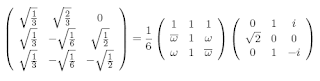where the second factor $V$ has the property that $V^{\dagger} V = 2 I$, for $I$ the identity matrix (the factor of 2 goes away with the appropriate normalisation factor). Let's have fun thinking about other properties of this operator!

## Friday, June 13, 2008

### M Theory Lesson 197

Speaking of platonic groups in neutrino physics, Lieven Le Bruyn beautifully clarifies the story in a post on Galois. As he points out, these three groups, the tetrahedral, octahedral and icosahedral,
in turn correspond to the three exceptional Lie algebras $E_6$, $E_7$, $E_8$ via the McKay correspondence (wrt. their 2-fold covers).
Yesterday we came across $\Gamma (3)$ in connection with the neutrino mixing tetrahedron. Recall that the generating function for $\Gamma (3)$ is $j^{\frac{1}{3}}$, where the dimension of $E_8$ appears in the second term of the expansion. But these connections to the exceptional Lie groups have much more to do with lattices and operads than with strings or toes, as Lieven promises to explain soon. M Theory is the theory that explains the structure of stringy geometry, not the theory that confirms so called stringy physics.

## Thursday, June 12, 2008

### M Theory Lesson 196

One place where the origin in the plane naturally appears in the theory of the modular group is in Reduction Theory, nicely explained in a paper recommended by Thomas Riepe. One relaxes the condition that the group action on the upper half plane shift the fundamental domain so that there is no intersection between the two domains, and allows a finite intersection. Then the region shown on the right,which is three times bigger than the usual domain, is allowed as a fundamental domain. Now let $\Gamma (N)$ be the congruence subgroup of the modular group. The translations of the new fundamental region give quotient spaces with punctures at vertices. For $\Gamma (3)$ one has a tetrahedron (like the neutrino tetrahedron), for $\Gamma (4)$ an octahedron and for $\Gamma (5)$ an icosahedron.

### M Theory Lesson 195

Recall from Mulase's lectures on the modular group $PSL (2, \mathbb{Z})$ that the generators are given by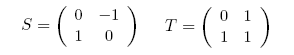where $T$ represents a translation by 1 in the complex plane. Note that $S$ really does square to the identity because $\pm 1$ are identified, but $T$ is quite distinct from the tetrahedral generator $(1, \omega , \omega^{2})$ used in neutrino mixing, which is more naturally associated with the quantum Fourier transform.

Consider how the diagonal $(\omega , \omega^{2})$ acts on $z$. As a modular transformation it would act via

$z \mapsto \frac{az + b}{cz + d} = \omega^{-1}z$

that is, a rotation by $\frac{2 \pi}{3}$ in the plane. This is like the action of $TS$, which also rotates a third of a circle but fixing instead the point $z = e^{\frac{\pi i}{3}}$ which is a vertex of the Grothendieck ribbon graph for the notorious j invariant.

## Monday, June 09, 2008

### Neutrinos Again III

Carl Brannen rightly pointed out that the matrices appearing in the tribimaximal mixing papers are in fact basically the same as those that characterise MUBs and the quantum Fourier transform for the prime 3. In fact, let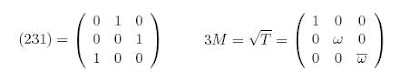where $(231)$ (oops, it should be $(312)$) denotes as usual the cyclic permutation in $S_{3}$ (sometimes drawn as ribbon diagrams) and $M$ is Carl's notation. Both $(231)$ and $3M$ cube to the identity. The democratic matrix is given by $D = \frac{1}{3}[1 + (231) + (231)^{2}]$, which can be thought of as a vector $(\frac{1}{3} , \frac{1}{3} , \frac{1}{3})$. Observe that the operator $S$ from the $A_{4}$ representation obeys the rules

$D \cdot 3S = 3D$
$2D - 3S = 3I$

where $I$ is the identity. I'm beginning to wonder if those poor experimenters are ever going to detect a $\theta_{13} > 0$. Note also that the (norm square) $2 \times 2$ form of the neutrino mass matrix, which was used by Harrison et al, is expressed as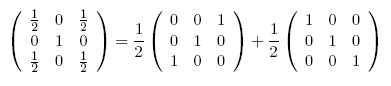which utilises the 2-circulant $3 \times 3$ matrix that happens to square to the identity. In other words, this is a $3 \times 3$ representation of the Pauli spin Fourier polynomial. Thus tribimaximal mixing is expressed as a composition of mass Fourier and spin Fourier components.

## Sunday, June 08, 2008

### Neutrinos Again II

Recall that a renormalised circulant matrix is a kind of magic square, where we don't worry about summing along diagonals. In neutrino physics, the unitarity of mixing forces the (squared) mixing matrix to be a magic square with rows and columns summing to 1. The tribimaximal case was first discussed by Harrison et al, where the whole matrix follows from the entries $U_{13}$, $U_{23}$ and $U_{12}$. Labelling columns by $\nu_{1}$, $\nu_{2}$, $\nu_{3}$ and rows by $e$, $\mu$, $\tau$ the matrix $U^{2}$ is

$\frac{2}{3}$ $\frac{1}{3}$ $0$
$\frac{1}{6}$ $\frac{1}{3}$ $\frac{1}{2}$
$\frac{1}{6}$ $\frac{1}{3}$ $\frac{1}{2}$

In terms of the standard mixing angles this corresponds to $\theta_{13} = 0$, $\theta_{23} = \frac{\pi}{4}$ and $\textrm{sin} \theta_{12} = \frac{1}{\sqrt{3}}$ with no additional (Dirac) CP violating phase. Given the excellent experimental agreement with this case, the question is, what is the justification for choosing $U_{13} = 0$, $U_{23} = \frac{1}{\sqrt{2}}$ and $U_{12} = \frac{1}{\sqrt{3}}$? Most physicists expect some deviation from tribimaximal mixing, but perhaps there is a good reason for things being so simple. For instance, observe that we can reorder the columns arbitrarily so that $U^{2}$ is derived (assuming one democratic column) from a diagonal

$\frac{2}{3}$, $\frac{1}{2}$, $\frac{1}{3}$

which is the length 3 Farey sequence. That is, it has the modular group property that for consecutive fractions $\frac{a}{b}$ and $\frac{c}{d}$, one has $bc - ad = 1$.

On the other hand, what mixing do we get if we substitute Carl's neutrino Koide rule for the one assumed by Harrison et al? Note that Harrison et al use the $3 \times 3$ circulant mass matrix for the charged leptons. On using the same quantum Fourier diagonalisation operator for both the charged leptons and neutrinos (see page 7 in Harrison et al) one would find that $U^{\dagger} U = 1$, so the tribimaximal mixing matrix would be replaced by the identity! It is the interplay of $3 \times 3$ circulants and $2 \times 2$ circulants that gives rise to the observed tribimaximal mixing.

## Saturday, June 07, 2008

### Neutrinos Again

A paper by Altarelli and Feruglio on tribimaximal mixing was mentioned in talks at Neutrino 08, so I thought I should take a look at it. They begin by describing the 3 dimensional representation of $A_{4}$ which has generators $S$ and $T$ satisfying $(ST)^{3} = S^{2} = 1$, just like the modular group, and also the relation $T^{3} = 1$. Then, letting $\omega$ be the cubed root of unity, one has for $T$ the matrix

1 0 0
0 $\omega^{2}$ 0
0 0 $\omega$

and for $3 S$ the circulant matrix

-1 2 2
2 -1 2
2 2 -1

The 12 elements of $A_{4}$ are given by all possible combinations of these generators. Gee, it already sounds a bit like M Theory. I can't imagine what they want with all the fairy fields and SUSY's mumbo jumbo, although in short shrift the tribimaximal mixing matrix appears.

## Friday, June 06, 2008

### M Theory Lesson 194

The denominator of the Fermi function is derived from the partition function

$Z = 1 + \textrm{exp}(- \frac{E - \mu}{kT})$

for the 2 possible occupancies of a fermion state, namely 0 or 1. A ternary analogue resulting in tripled Pauli statistics would require

$Z = 1 + 3 \textrm{exp} (- \frac{E}{kT})$

where we arbitrarily shift the energy scale, momentarily. Presumably this corresponds to the three possible ways of occupying the state with one particle, whereas for ordinary fermions there is only one way of occupying a state. Another interpretation is to write

$3 \textrm{exp} (- \frac{E}{kT}) = \textrm{exp} (- \frac{E}{kT} + \textrm{log} 3)$

where $\textrm{log} 3$ is an energy level (for one prime object) in the Riemann gas system, whose complete partition function is the Riemann zeta function $\zeta (s)$ for $s = (kT)^{-1}$.

## Thursday, June 05, 2008

### POW Riemann II

A more respectable result using Riemann zeta values is

$(\zeta (2) - 1) + (\zeta (3) - 1) + (\zeta (4) - 1) + \cdots = 1$

because the terms in this series start at 0.6449 and rapidly approach zero. It is well known that for even ordinals

$\zeta (2k) = \frac{(-1)^{k+1} (2 \pi)^{2k}}{2 (2k)!} B_{2k}$

for Bernoulli numbers $B_{2k}$. More recently, formulas for odd ordinals have been found by Linas Vepstas. From his 2006 paper we have

$\zeta (4m - 1) = - 2 \sum_{n} \textrm{Li}_{4m - 1} (e^{- 2 \pi n}) - \frac{1}{2} (2 \pi)^{4m - 1} \sum_{j=0}^{2m} (-1)^{j} \frac{B_{2j} B_{4m - 2j}}{(2j)!(4m - 2j)!}$

$\zeta (4m + 1) = (1 + (-4)^{m} - 2^{4m + 1})^{-1} [-2 \sum_{n} \textrm{Li}_{4m + 1} (e^{- 2 \pi n + \pi i})$
$+ 2(2^{4m+1} - (-4)^{m}) \sum_{n} \textrm{Li}_{4m + 1} (e^{- 2 \pi n})$
$+ (2 \pi)^{4m+1} \sum_{j=0}^{m} (-4)^{m+j} \frac{B_{4m - 4j + 2}B_{4j}}{(4m - 4j + 2)!(4j)!}$
$+ \frac{1}{2} (2 \pi)^{4m+1} \sum_{j=0}^{2m+1} (-4)^{j} \frac{B_{4m - 2j + 2}B_{2j}}{(4m - 2j + 2)!(2j)!} ]$

for $\textrm{Li}_{s}(x)$ the polylogarithm function, which generalises the Riemann zeta function. In other words, one can think of $\zeta (4m - 1)$ as the $n = 0$ term in a formula relating polylogarithm values to the Bernoulli numbers.

## Wednesday, June 04, 2008

### POW Riemann

Todd and Vishal's Problem of the Week number 3 (solution here) was to compute, for any $n > 1$, the series (from $k = 0$)

$S(n) \equiv \sum_{k} B(n+k ; k)^{-1}$

where $B(n+k ; k)$ is a binomial coefficient. In the case $n = 2$ we see that the sum takes the form

$S(2) = 1 + \frac{1}{3} + \frac{1}{6} + \frac{1}{10} + \cdots = 2$

which is a sum of reciprocals of triangular numbers $\frac{1}{2} k(k+1)$ (from $k = 1$). For $n = 3$ we obtain the reciprocals of the tetrahedral numbers, and $S(3) = \frac{3}{2}$. The tetrahedral number $T_{k} = \frac{1}{6} k(k+1)(k+2)$ is the sum of the first $k$ triangular numbers. By the way, only three tetrahedral numbers are perfect squares, namely 1, 4 and $T_{48} = 19600$. One guesses that in general $S(n)$ is a series of reciprocals of tetrahedral numbers in dimension $n$. Indeed

$S(n) = \frac{n}{n - 1}$

But whenever discussing infinite series of simple polytopes, an M theorist cannot help thinking of the Riemann zeta function. Observe that for $n = 2$

$S(2) = \sum_{k} \frac{2}{k^{2} + k} = 2 \zeta (2) - \sum_{k} \frac{2}{k^{3} + k^{2}}$
$= 2 [ \zeta (2) - \zeta (3) + \zeta (4) - \zeta (5) + \cdots ] = 2$

from which one deduces, allowing cancellation of infinities (!), that

$\zeta (2) - \zeta (3) + \zeta (4) - \zeta (5) + \cdots = 1$

What kind of zeta sums do we get in general?

## Tuesday, June 03, 2008

### F Theory

Since my brief comment regarding the new F Theory paper was naturally deleted by Woit, although quite evidently very much on topic, I will post it here:

Hmmm, that’s odd. A 200 page paper on F theory should be able to recover Brannen’s precise mass values for the three Standard Model neutrinos ($\sum m_{i}$ = 0.06 eV) because the 12 dimensions are recovered very simply from the three Riemann moduli spaces of twistor dimension (= 6 over $\mathbb{R}$) via marked points = spatial dimension and also holes = times (one hole for the torus and two for the genus two surface).

## Sunday, June 01, 2008

### M Theory Lesson 193

While I was busy at Neutrino 08, the NCG blog posted an update on the Vanderbilt meeting. In particular, they note that Manin's lectures on Zeta functions and Motives are available at Katia Consani's homepage! Niranjan Ramachandran spoke about this paper at Vanderbilt. This work, originating in the physical ideas of Deninger, looks at the field over one element (which is fast becoming a popular subject). Deninger writes the zeta function, completed with the infinite prime, in the form

$\zeta (s) = \frac{R}{s (s - 1)}$

where $R$ is a regularized determinant to be viewed as an infinite dimensional analogue of a determinant of an endomorphism of a finite dimensional vector space (according to Connes and Consani).

### Neutrino08 - Sarkar

Subir Sarkar is one of those rare individuals who can be very critical of his audience without offending anybody (that I know of) because he just makes so much sense. He was assigned the task (by the organisers) of analysing the implications of cosmic ray results. The talk included phrases such as:
one could use some Mickey Mouse model of mirror [something] ...
or even better, to the audience:
please stop drawing limits ...
in reference to ideas which have been completely ruled out. The talk began with the catchphrase guaranteed cosmogenic neutrino flux, to which Sarkar had added strong quotation marks to the first word, the main point being that cosmic ray primaries might well be heavy nuclei rather than protons. Auger data was used as evidence for this hypothesis, which is consistent with the observed energy spectrum and predicts a smaller cosmogenic flux.

Another 2006 paper looks at Auger bounds for QCD. Colour gluon condensates were mentioned. The steep rise of gluon density at low x should saturate, leading to a supression of the neutrino-nucleus cross section. In summary, he says that neutrino observations are a unique laboratory for both Standard Model and Beyond Standard Model physics.

### Neutrino08 Day 6c

ANITA is a radio balloon experiment, which is flown around Antarctica to view a vast expanse of ice and then (ideally) landed neatly. Despite problems with the last flight, 18 days of good live time were recovered for an average 1.2 km depth of ice. For the next flight they expect a factor of 5 improvement in the $\nu$ rate. Some candidate geosynchrotron events were observed, but satellite data still needs to be checked carefully (solid state relays on satellites can cause false events).

B. Dingus overviewed multiwavelength astronomy and, taking advantage of the late lecture slot, showed a few photos of her trip to Franz Josef glacier (and Arthur's Pass).Potential neutrino sources were introduced with this stunning image of the Crab nebula, along with other examples. Unidentified high latitude EGRET sources were also mentioned. And GLAST is due to launch on Thursday! One day of GLAST operation should match 9 years of EGRET. Dingus actually works for Milagro, a TeV gamma ray observatory in Mexico that was turned off in April 2008. HAWC was discussed as a promising future detector.

### Neutrino08 Day 6b

E. Roulet reported from the Pierre Auger Cosmic Ray Observatory in Argentina. This consists of 1600 detectors spread over 3000 $\textrm{km}^{2}$ along with 24 telescopes looking at the sky over the region. Pierre Auger recently confirmed the infamous GZK cutoff (more on this later) associated to proton energies greater than $6 \times 10^{19}$ eV. Rather, although high energy events were observed, the flux falls off by about a half with more than $6 \sigma$. High energy events are extragalactic. For 2006-2007 data, 8 strong correlations between events and nearby AGN were found, as compared to 3 expected. Centaurus A (the closest AGN) corresponds to 2 events located within 3 degrees. No candidates for diffuse neutrino flux were observed. Construction of Pierre Auger is almost completed.

### Neutrino08 Day 6a

Everyone who goes down to Antarctica to work on IceCube must pass through Christchurch, and it is not surprising that UC has a neutrino physics group, which is led by Jenni Adams. The IceCube talk on Saturday morning was given by S. Klein, who began with a description of the detector: 4800 optical modules on 80 strings reaching down 2450m into the ice at the South Pole. This depth represents roughly 100000 years of atmospheric history, and one can see ancient volcanic eruptions due to dust layers observed in the calibration data. There is also a 1 $\textrm{km}^{2}$ surface array of tanks. When the full detector (IC80) is in operation in 2011 they estimate 200 $\nu$ events per day.

There are a number of trigger systems, for example, the firing of 5 of 7 adjacent optical modules on a single string within $1.5 \mu\textrm{s}$. About 6% of events are considered sufficiently interesting to send north via satellite. IceCube is close to releasing a skymap for IC22, which is about 5 times more sensitive than IC9. Some searches were triggered, including the very bright GRB080119B event, although IceCube only expects 0.1 associated $\nu_{\mu}$ events.

Results: The solar WIMP search found no excess and the AMANDA limits have been improved. The solar outburst of December 13, 2006 indicated no large spectral changes. A preliminary cosmic ray spectrum was shown.